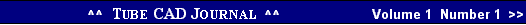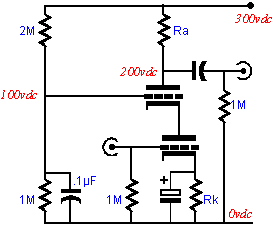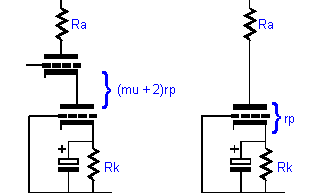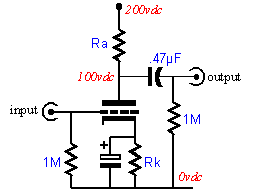High Transconductance    In the Grounded Cathode amplifier the transconductance of the triode is mitigated by the addition of the plate resistor. Ra when summed with the plate resistance and divided into the mu of the triode, yields the resulting transconductance:           Gm = mu / (rp + Ra).    This decrease in transconductance reduces the potential gain of the amplifier. In the Cascode amplifier, on the other hand, the bottom triode's transconductance is nearly identical to its static value. The resistance R presented at its plate is equal to the top triode's rp added to the plate resistor's value divided by its mu:            R = (rp + Ra) / mu. The result of this resistance R in parallel with the rp of the bottom triode divided into the mu of the triode yields the resulting transconductance:           Gm = mu / (rp + R).
 Improving the Cascode's PSRR
 Review of the Cascode's Operation   The Cascode is a compound amplifier. One triode stands on top of another, while sharing a common current path. The top triode strives both to shield the input grid from the top triode's Miller effect and to preserve the transconductance of the bottom triode. The result is amplifier with both high gain and extended bandwidth.Textbook Cascode Amplifier
 Low Input Capacitance    In the Grounded Cathode amplifier, the grid-to-plate capacitance is multiplied by the gain that the triode realizes working into its plate resistor. This effective increase in capacitance greatly reduces the high frequency response of the amplifier; whereas in the Cascode amplifier, the input grid-to-plate capacitance is virtually identical to its static value, as its plate voltage is held at a nearly constant value.
 Cascode & Grounded Cathode Amplifiers
 Cascode VS Grounded Cathode   So far, in terms of transconductance and low input capacitance, the Cascode seems like a clear winner. Where it falls short is in its high output impedance and a near zero PSRR. With the Grounded Cathode amplifier, the output impedance is equal to Ra || rp; with the Cascode, the output impedance is equal to Ra || (mu + 2) rp. For example, a 6DJ8 used in a Grounded Cathode amplifier with a 9K plate load resistor and a bypassed cathode resistor, will have a Zo at its output  of 2,250 ohms:            Zo = Ra || rp           Zo = 9,000 || 3,000           Zo = 2,250When the 6DJ8 is used in a Cascode circuit, with the same 9K plate resistor, the math look like this:           Zo = Ra || (mu + 2) rp           Zo = 9,000 || (33 + 2) 3,000           Zo = 8,270.    The Grounded Cathode amplifier achieves a respectableTextbook Grounded Cathode Amplifier2 pg.